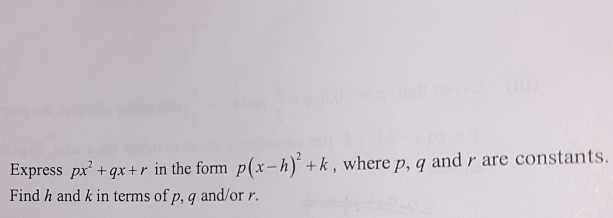# Questionpx2 + qx + r =  p (x – h)2 + k

= p(x2 -2hx + h2 ) + k

=px2 -2phx + (ph2 + k)

So comparing the coefficient of x,

q = -2ph   =>  h = -(q/2p)

Comparing the constant,

r = ph2 + k     but we know  h = -(q/2p) from above,

So r = p[ -(q/2p) ]2 + k

r   = p [ (q2 /4p2 ) ] + k

Therefore,  k = r – (q2 /4p)

0 Replies 2 Likes ✔Accepted Answer